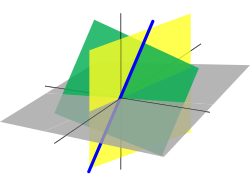# Data Science Fundamentals

Filter

The field of Data Science has been growing exponentially over the past several years, to the point that now it is inescapable. Even for those who are not looking to become data scientists, the need to understand how analytical insights are gained from data is becoming a job requirement. With so much data-driven decision making, understanding the foundations of this field is a good addition to anyone's skill set.

This track focuses on learning the underpinnings of the field of data science. This includes the statistical and mathematical concepts that inform Machine Learning algorithms, and that power the decisions of companies around the globe. Through these courses, you will see the foundational pieces of data science, including:

Statistics & Probability• Understand probability theory
• Interpretation of bayesian distribution
• Different types of probability frequency distributions
Linear Algebra Fundamentals• Vectors and matrices
• Matrix operations
• Eigen values
• Eigen vectors
An introduction to calculus of data science models• Differential Calculus
• Integral Calculus
• Multivariate Calculus
• Numerical methods

This track is meant for beginners to the field of data science, and to programming or software in general. At least a basic understanding of algebra is a benefit, and completion of high school math at least will make you more likely to understand the concepts, though anyone who's an analytical thinker should be able to get something useful out of these courses.

4 Classes

4 Data Science Fundamentals